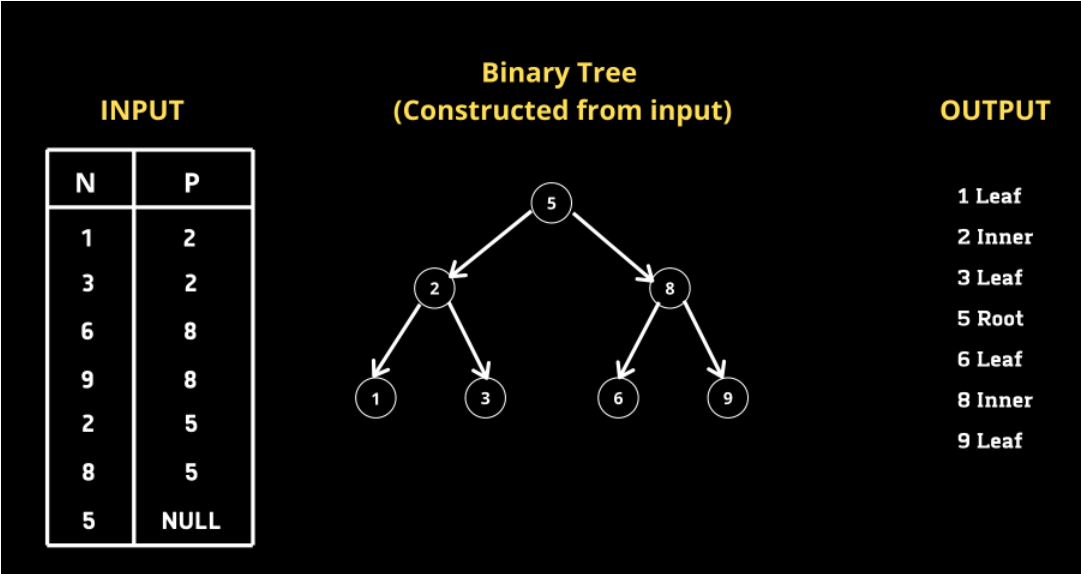# Binary Tree Node in SQL

Most of us have already solved some Binary Tree-related questions in Data Structure and Algorithms. But have you ever tried to solve the Binary Tree Question in SQL?

Today with this blog let's give a try to solve the Binary Tree Node Problem in SQL.

## Problem Statment:-

We are given a table, which is a Binary Tree consisting of two columns Node and Parent. We must write a query that returns the node type ordered by the value of nodes in ascending order.

Each node in the tree can be one of three types:

• "Leaf": if the node is a leaf node.
• "Root": if the node is the root of the tree.
• "Inner": If the node is neither a leaf node nor a root node.

Write an SQL query to report the type of each node in the tree. Return the result table ordered by `id` in ascending order.

The query result format is in the following example.## My Solution:-

Before Jumping into the solution I would suggest you to solve this question yourself first and then jump to the final solution.

Upon initial analysis, we can conclude that if a given node N has its corresponding P-value as NULL it is the root. And for a given Node N if it exists in the P column it is not an inner node. Based on this idea let us write a query.

``````SELECT CASE
WHEN P IS NULL THEN 'Root'
WHEN N IN (SELECT DISTINCT P from TreeNode) THEN 'Inner'
ELSE 'Leaf'
END AS Type
FROM TreeNode
ORDER BY N ASC;We can use CASE statement which acts as a switch function. As I mentioned if P is NULL for a given node N then N is the root. Similarly, if a given node N is in column P it is an inner node. To get all nodes from column P we wrote a subquery which returns all the distinct nodes in column P. Since we were asked to order the output by node values in ascending order we used the ORDER BYClause.Thanks for reading. I hope you enjoyed this Tree Node Problem - it was fun for me to create!. You can also share your approach to solve this problem.``````